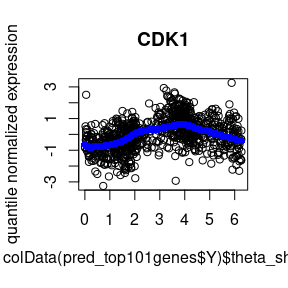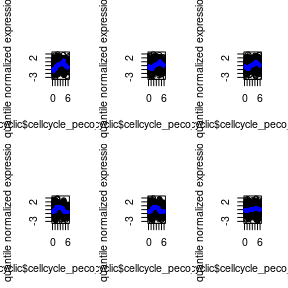Predicting cell cycle phase using peco

Overview

peco is a supervised approach for PrEdicting cell cycle phase in a COntinuum using single-cell RNA sequencing data. The R package provides functions to build training dataset and also functions to use existing training data to predict cell cycle on a continuum.

Our work demonstrated that peco is able to predict continuous cell cylce phase using a small set of cylcic genes: CDK1, UBE2C, TOP2A, HISTH1E, and HISTH1C (identified as cell cycle marker genes in studies of yeast (Spellman et al., 1998) and HeLa cells (Whitfield et al., 2002)).

Below we provide two use cases. Vignette 1 shows how to use the built-training dataset to predict continuous cell cycle. Vignette 2 shows how to make a training datast and build a predictor using training data.

Users can also view the vigenettes via browseVignettes("peco").

training_human stores built-in training data of 101 significant cyclic genes. Below are the slots contained in training_human:

• predict.yy: a gene by sample matrix (101 by 888) that stores predict cyclic expression values.
• cellcycle_peco_reordered: cell cycle phase in a unit circle (angle), ordered from 0 to 2$$pi$$
• cellcycle_function: lists of 101 function corresponding to the top 101 cyclic genes identified in our dataset
• sigma: standard error associated with cyclic trends of gene expression
• pve: proportion of variance explained by the cyclic trend
data("training_human")

Predict cell cycle phase using gene expression data

peco is integrated with SingleCellExperiment object in Bioconductor. Below shows an example of inputting SingleCellExperiment object to perform cell cycle phase prediction.

sce_top101genes includes 101 genes and 888 single-cell samples and one assay slot of counts.

data("sce_top101genes")
assays(sce_top101genes)
#> List of length 1
#> names(1): counts

Transform the expression values to quantile-normalizesd counts-per-million values. peco uses the cpm_quantNormed slot as input data for predictions.

sce_top101genes <- data_transform_quantile(sce_top101genes)
#> computing on 2 cores
assays(sce_top101genes)
#> List of length 3
#> names(3): counts cpm cpm_quantNormed

Apply the prediction model using function cycle_npreg_outsample and generate prediction results contained in a list object pred_top101genes.

pred_top101genes <- cycle_npreg_outsample(
Y_test=sce_top101genes,
sigma_est=training_human$sigma[rownames(sce_top101genes),], funs_est=training_human$cellcycle_function[rownames(sce_top101genes)],
method.trend="trendfilter",
ncores=1,
get_trend_estimates=FALSE)

The pred_top101genes$Y contains a SingleCellExperiment object with the predict cell cycle phase in the colData slot. head(colData(pred_top101genes$Y)$cellcycle_peco) #> 20170905-A01 20170905-A02 20170905-A03 20170905-A06 20170905-A07 20170905-A08 #> 1.099557 4.680973 2.356194 4.303982 4.052655 1.413717 Visualize results of prediction for one gene. Below we choose CDK1 (“ENSG00000170312”). Because CDK1 is a known cell cycle gene, this visualization serves as a sanity check for the results of fitting. The fitted function training_human$cellcycle_function[] was obtained from our training data.

plot(y=assay(pred_top101genes$Y,"cpm_quantNormed")["ENSG00000170312",], x=colData(pred_top101genes$Y)$theta_shifted, main = "CDK1", ylab = "quantile normalized expression") points(y=training_human$cellcycle_function[["ENSG00000170312"]](seq(0,2*pi, length.out=100)),
x=seq(0,2*pi, length.out=100), col = "blue", pch =16)Visualize cyclic expression trend based on predicted phase

Visualize results of prediction for the top 10 genesone genes. Use fit_cyclical_many to estimate cyclic function based on the input data.

# predicted cell time in the input data
theta_predict = colData(pred_top101genes$Y)$cellcycle_peco
names(theta_predict) = rownames(colData(pred_top101genes$Y)) # expression values of 10 genes in the input data yy_input = assay(pred_top101genes$Y,"cpm_quantNormed")[1:6,]

# apply trendfilter to estimate cyclic gene expression trend
fit_cyclic <- fit_cyclical_many(Y=yy_input,
theta=theta_predict)
#> computing on 2 cores

gene_symbols = rowData(pred_top101genes$Y)$hgnc[rownames(yy_input)]

par(mfrow=c(2,3))
for (i in 1:6) {
plot(y=yy_input[i,],
x=fit_cyclic$cellcycle_peco_ordered, main = gene_symbols[i], ylab = "quantile normalized expression") points(y=fit_cyclic$cellcycle_function[[i]](seq(0,2*pi, length.out=100)),
x=seq(0,2*pi, length.out=100), col = "blue", pch =16)
}